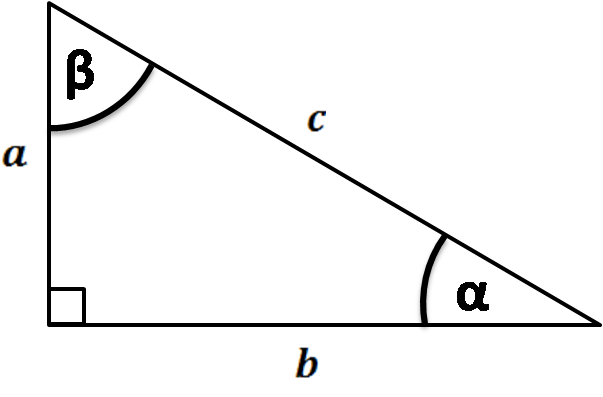# Tangent Ratio Calculator

Created by Davide Borchia
Reviewed by Anna Szczepanek, PhD
Last updated: Jun 05, 2023

Learn how to calculate the tangent ratio in a right triangle with this handy tool. Discover the relationship between the tangent trigonometric function and triangles, how to find (when possible) the tangent ratio, and much more!

## What is the tangent ratio?

The tangent ratio is a way to define the trigonometric function tangent in right triangles* — and not only. We define the tangent ratio as a way to calculate the tangent of an angle when the angle itself is unknown.

To define the tangent ratio, we need to consider a triangle, a right triangle to be precise. In it, we identify:

• Two acute angles (say, $\alpha$ and $\beta$);
• Two sides that form a right angle at their intersection, the legs of the triangle; and
• A right angle and the hypotenuse opposite to it.

Each angle is associated with an opposite and an adjacent leg, and their roles switch when we consider the other angle. The opposite leg is the one with which the angle doesn't share a side, while the adjacent one corresponds to one of the angles' sides. Now that you identified the elements of a right triangle, we can answer the question: "what is the tangent ratio?.In the figure we identify the angle α\alphaα, with oppostie leg aaa and adjacent leg bbb, and the angle β\betaβ with opposite leg bbb and adjacent leg aaa.

The tangent ratio is the ratio between the opposite and the adjacent leg. This quantity also corresponds to the tangent of the angle we used in identifying the legs. Writing this as a formula, we have:

$\tan(\alpha)=\frac{\mathrm{opposite}}{\mathrm{adjacent}}$

From this simple formula, we can already point out some features of the tangent function:

• When the adjacent leg goes to $0$, the tangent is undefined. This condition corresponds to what we find when the angle $\alpha$ is equal to $90\degree$.
• Since the tangent ratio is defined within right triangles, we also notice that it can only assume positive values: this tells us that we only need to know the results for angles between $0\degree$ and $90\degree$ to figure out all the others around the circle.

## Tangent ratio and the Pythagorean theorem

To calculate the tangent ratio, you must know the length of the legs of the right triangle you're working on. If you don't know the length of a leg, use the Pythagorean theorem to obtain it from the hypotenuse.

Once you know the length of the legs, calculate both the ratios: your results are the tangents of the two acute angles of the right triangle. If you want to calculate the angles, use the inverse tangent function!

## FAQ

### What is the tangent ratio?

The tangent ratio is a quantity defined in right triangles equal to the tangent of an acute angle and calculated through the ratio of the length of the legs (the catheti) of the triangle. You can always find two tangent ratios in each triangle. The value of the tangent ratio is between 0 (included) and ∞ (excluded).

### How do I calculate the tangent ratio in a right triangle?

To calculate the tangent ratio in a right triangle:

1. Identify the angle for which you want to calculate the tangent ratio.
• Measure the opposite leg.
2. Calculate the ratio between the opposite and the adjacent leg. The result is the tangent ratio:
tangent ratio = opposite/adjacent.
3. Since the tangent ratio is equal to the tangent of the acute angle, you can use the inverse tangent function to find the value of the angle itself.

### What is the tangent ratio is a 3-4-5 right triangle?

You can identify two tangent ratios in a 3-4-5 triangle. To find them:

1. Identify the legs of the right triangle: they have lengths 3 and 4. Let's call them a and b.
2. Calculate the two possible ratios:
• r1 = a/b = 3/4; and
• r2 = b/a = 4/3.
3. The values of the ratios are also the tangents of the two acute angles in the right triangle. We can use the inverse tangent to find the following:
• α = arctan(3/4) = 36.87°; and
• ß = arctan(4/3) = 52.13°;
Davide Borchia
Opposite (a)
Tangent
Angle (α)
deg
People also viewed…

### Cylinder volume

The cylinder volume calculator helps in the calculation of the right, oblique and hollow cylinder volume.

### Free fall

Our free fall calculator can find the velocity of a falling object and the height it drops from.

### Podcasts

Do you feel like you could be doing something more productive or educational while on a bus? Or while cleaning the house? Well, why don't you dive into the rich world of podcasts! With this podcast calculator, we'll work out just how many great interviews or fascinating stories you can go through by reclaiming your 'dead time'!